# Eric “EZ” Zuley & Jose Beltran Birthday Party 2016 at the H.O.M.E. in Beverly Hills

<p< < p=””>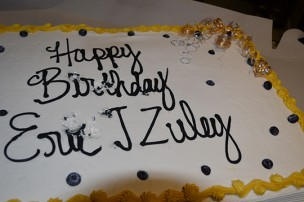<p< < p=””> </p< <>

<p< < p=””>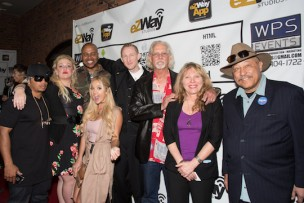<p< < p=””> </p< <>

Celebrity photo credit: Sheri Determan <p< < p=””> </p< <>

<p< < p=””> <p< < p=””>

</p< <></p< <></p< <>

<p< < p=””> <p< < p=””>

<p< < p=””>

Teen actress singer Laci Kay for Actors Reporter performed at and covered the Eric “EZ” Zuley, Jose Beltran Birthday Celebration at H.O.M.E. Beverly Hills red carpet. <p< < p=””> </p< <>

<p< < p=””>

There were special appearances by: Judge Joe Brown, Choreographer Shane Sparks (ABDC Judge/Master P Show), Munni Irone (Founder A4PAwards) Carmelita Pittman (singer, songwriter, producer, and Founder of The Rose Breast Cancer Society.), Michael Zanetis (Executive Producer BB KING Film), Mary Desmond (American Idol), Shevyn Roberts (Opened for Justin Bieber), Laci Kay (Modern Family), Vincent M. Ward (Walking Dead), Trae Ireland (Actor), Patrick Faucette (Own Tyler Perry The Have and the have nots), Reatha Grey (Betty Whites Off Their Rockers), Ken Davitian (Borat), Kaye-Cee Austin (Founder TeenTrep.Co), Faithy J (CEO TeenTrep.Co), Kate Linder (Hollywood Walk Of Fame), and David Eugene Andrews (Author of The English Slave), Jay Pugh (RK Records), Rosalyn Kahn (author and TedX Speaker) Penny Lambright (founder Patriots and Paws), Lalanii R. Grant (Creative Writer), Tori V (Actress), Ewart Chin (Actor), Jonathan JG Goldstein (James Brown’s right hand man) and many others.

<p< < p=””>

<p< < p=””>

Celebrities and fans were entertained by singer performers Laci Kay, FaithyJ, Shevyn Roberts and the celebrity magician Evan Disney displaying his amazing skills, Allen Ramos, Lili Lev, Mary Desmond (American Idol). <p< < p=””> </p< <>

There were be raffles and numerous other goody giveaways, as well as auctions and opportunities for charity donations.
Acknowledge were United States Veterans, who have served and fought for our country. The evening activities included three Expert Panels and guests were allowed to participate in the amazing Q&A sessions. <p< < p=””> </p< <>

As DJ Juan played a selection of soothing music in the background, this became truly a night to be remember. <p< < p=””> </p< <>

<p< < p=””>

The evening also included: <p< < p=””> </p< <>

Launch of the new Waz-Kal Verizon, Amazon eZWayTV Channel. <p< < p=””> </p< <>

and <p< < p=””> </p< <>

2 screenings:
1. Mr. & Mrs. Smith The Series Trailer
and
2. Wind International Film Festival

<p< < p=””>

<p< < p=””>

Photos by EZ Broadcasting: <p< < p=””> </p< <>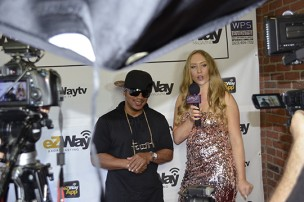<p< < p=””> </p< <>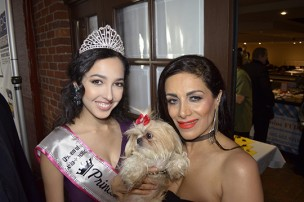<p< < p=””> </p< <>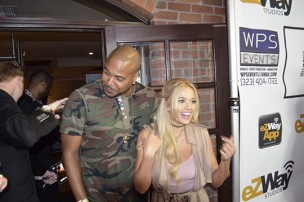<p< < p=””> </p< <>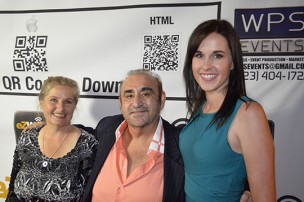<p< < p=””> </p< <>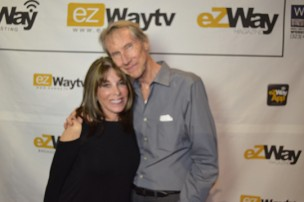<p< < p=””> </p< <>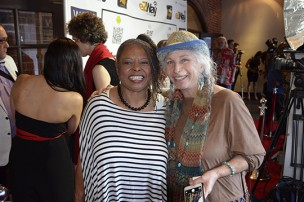<p< < p=””> </p< <><p< < p=””> </p< <>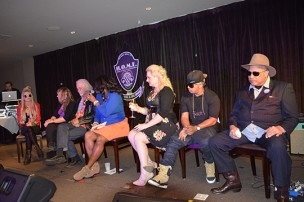<p< < p=””> </p< <>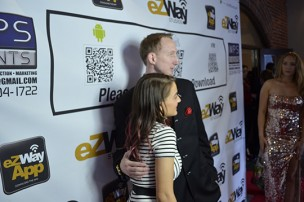<p< < p=””> </p< <>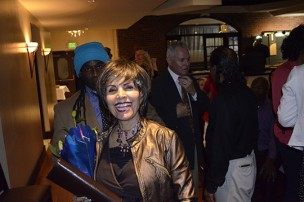<p< < p=””> </p< <>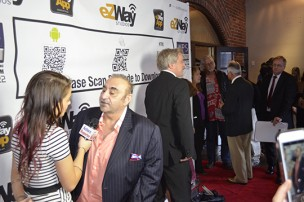<p< < p=””> </p< <>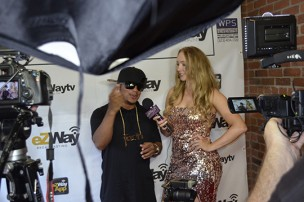<p< < p=””> </p< <>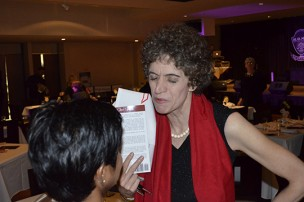<p< < p=””> </p< <>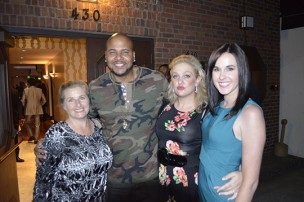<p< < p=””> </p< <>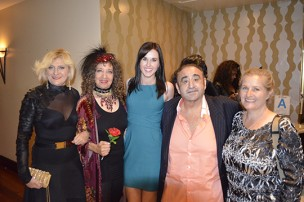<p< < p=””> </p< <>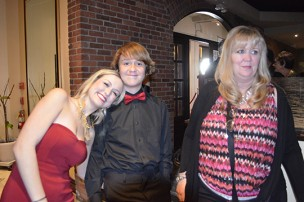<p< < p=””> </p< <>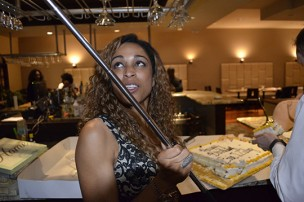<p< < p=””> </p< <>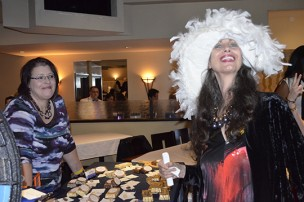<p< < p=””> </p< <>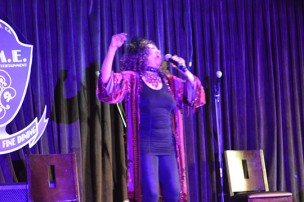<p< < p=””> </p< <>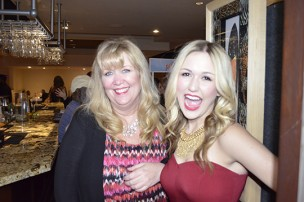<p< < p=””> </p< <>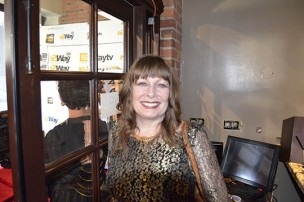<p< < p=””> </p< <>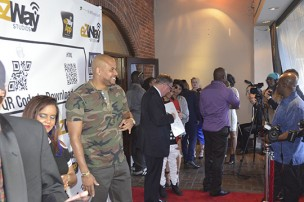<p< < p=””> </p< <>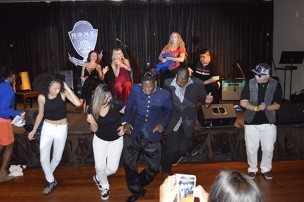<p< < p=””> </p< <>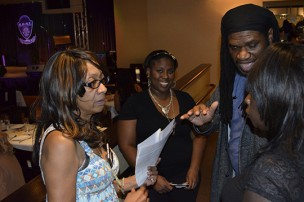<p< < p=””> </p< <>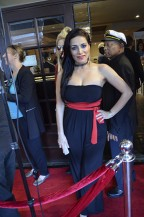<p< < p=””> </p< <>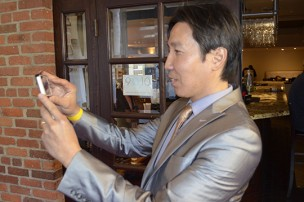<p< < p=””> </p< <>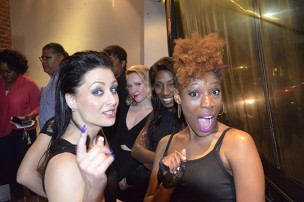<p< < p=””> </p< <>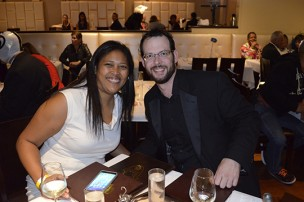<p< < p=””> </p< <>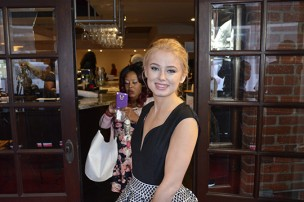<p< < p=””> </p< <>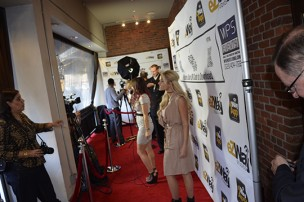<p< < p=””> </p< <>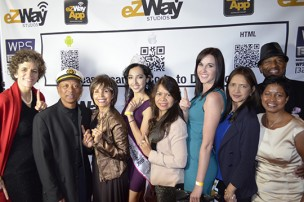<p< < p=””> </p< <>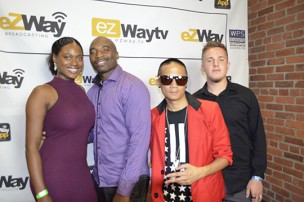<p< < p=””> </p< <>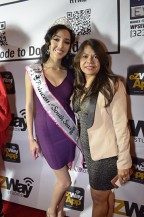<p< < p=””> </p< <>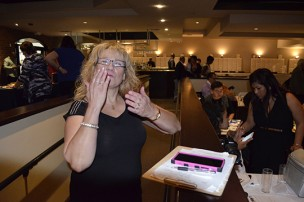<p< < p=””> </p< <>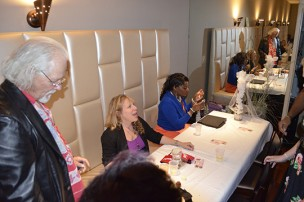<p< < p=””> </p< <>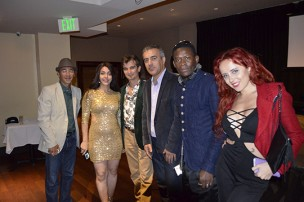<p< < p=””> </p< <>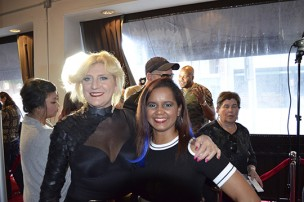<p< < p=””> </p< <>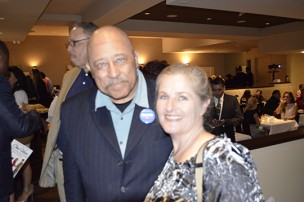<p< < p=””> </p< <>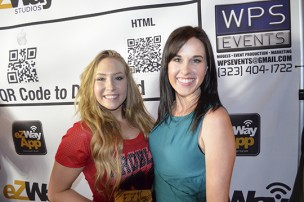<p< < p=””> </p< <>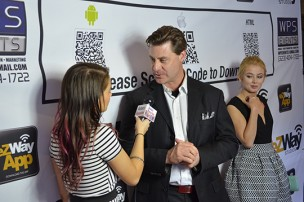<p< < p=””> </p< <>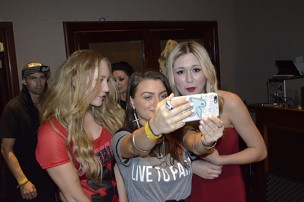<p< < p=””> </p< <>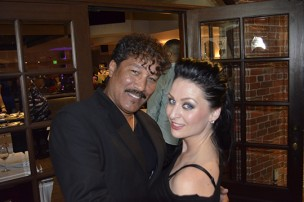<p< < p=””> </p< <>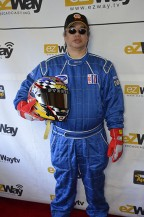<p< < p=””> </p< <>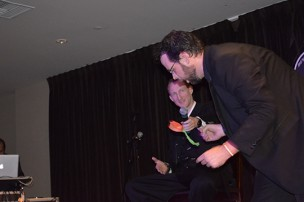<p< < p=””> </p< <>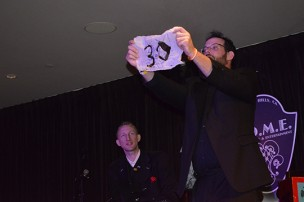<p< < p=””> </p< <>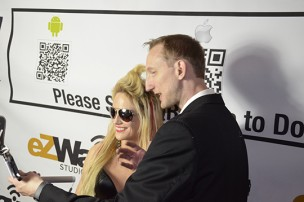<p< < p=””> </p< <><p< < p=””> </p< <>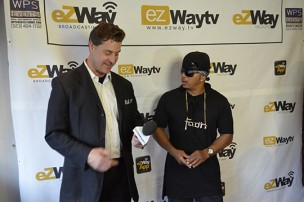<p< < p=””> </p< <>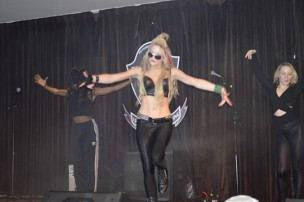<p< < p=””> </p< <>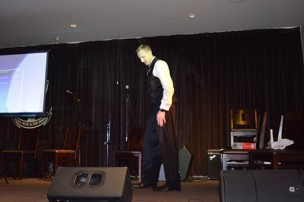<p< < p=””> </p< <><p< < p=””> </p< <>

<p< < p=””>

The evening was produced by: eZWay Broadcasting, INC and WPS Events. <p< < p=””> </p< <>

<p< < p=””>

This event was sponsored by Get Jazzed Munchies (Jennifer A. Zaft), WPS Events, TeenTrep.Co, W.e.i, The Rose Breast Cancer Society, and Pike & Sons Fudge (Renee Pike, Dillon Pike, Zachary Pike Pike and Sons. <p< < p=””> </p< <>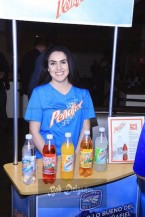<p< < p=””> </p< <>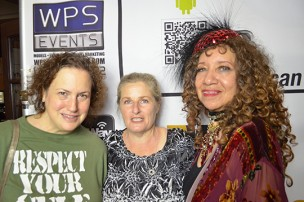<p< < p=””> </p< <>

<p< < p=””> <p< < p=””> </p< <></p< <>

<p< < p=””>

H.O.M.E. in Beverly Hills is one of the most popular locations, where people like Stevie Wonder and other A-List celebrities have performed. The food and service at this well-known location has received amazing ratings. This event at the House of Music and Entertainment
(HOME90210.com) will feature seven different, talented music artists, performing in a variety of music genres. <p< < p=””> </p< <>

<p< < p=””>

Laci Kay Official <p< < p=””> </p< <>

<p< < p=””>

And, Thank you to Bob Delgadillo Events Photographer

and Thank you to: Sheri Determan Photography

<p< < p=””>

<p< < p=””>

Eric “EZ” Zuley’s birthday party at H.O.M.E. in Beverly Hills, coverage by Actors Reporter, a channel on the Actors Podcast Network, a Pepper Jay Production.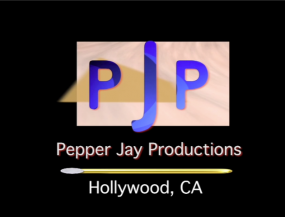<p< < p=””>

<p< < p=””></p< <></p< <></p< <></p< <></p< <></p< <></p< <></p< <></p< <></p< <></p< <></p< <></p< <></p< <></p< <></p< <></p< <></p< <></p< <>